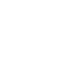Course cost: \$300 / month
Course duration: 1 month, 32 hours
Course start date: September 23, 10:00 - 13:00, Saturdays
Course entry requirements: any programming language experience

*The course is available in the following languages: English, Russian, Ukrainian
Algorithms for
programmers
Course program
Algorithm complexity estimation
• Basic denotations. Asymptotic complexity estimation. Classification of algorithms.
Sort algorithms
• Bubble sort. Insertion sort. Quick sort. Merge sort. Heapsort. Complexity estimation of sort algorithms.
Basic data structures
• Singly linked list. Doubly linked list. Queue. Stack. Basic lists operations implementation.
Trees
• Binary trees. Balanced and unbalanced trees (B-trees). Tree representation as an array and as a linked structure. Recursive and non-recursive tree traversal algorithms. Binary search AVL tree. Red-black tree. An example of using trees: the Huffmann algorithm.
Dynamic programming
• Basic algorithms of dynamic programming.
Greedy Algorithms
• Coin exchange problem. Knapsack problem.
Graphs
• The concept of a graph. Graph representation. Connected and disconnected graphs. Connectivity components. Spanning tree. Graphs applications.
Basic Graph Algorithms
• Breadth-first search. Finding the shortest path (Dijkstra's algorithm). Depth-first search. Topological sorting. Finding bridges in a graph. Loops in a graph.
Genetic Algorithms
• Usage and implementation of genetic algorithms.
Elements of cryptography
• RSA algorithm. ElGamal algorithm. Attacks on cryptographic algorithms.
Parallel programming